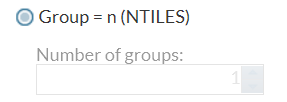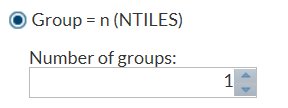# Example 3: Selecting a Radio Button Enables a Number Stepper Control

In this example from the Rank Data task, the selection of the Group = n (NTILES) option determines whether the Number of groups option is available. (In the Tasks section, the Rank Data task is in the Data group.)
In this example, RMGN is the name of the radio button, and NUMGRPS is the name of the `numstepper` variable.
```<Option name="RMGN" inputType="radio" variable="RMGRP">Group = n (NTILES)</Option>
<Option name="NUMGRPS" defaultValue="1" minValue="0" inputType="numstepper"
indent="1">Number of groups:</Option>

<Dependency condition="\$RMGRP.equalsIgnoreCase('RMGN')"/>
<Target conditionResult="true" option="NUMGRPS" action="enable"/>
<Target conditionResult="false" option="NUMGRPS" action="disable"/>
</Dependency>
```
By default, the Group = n (NTILES) option is not selected, so the Number of groups option is not available. Here is the result that appears on the Options tab:When you select the Group = n (NTILES) option, the Number of groups option is available. Here is the result that appears on the Options tab: